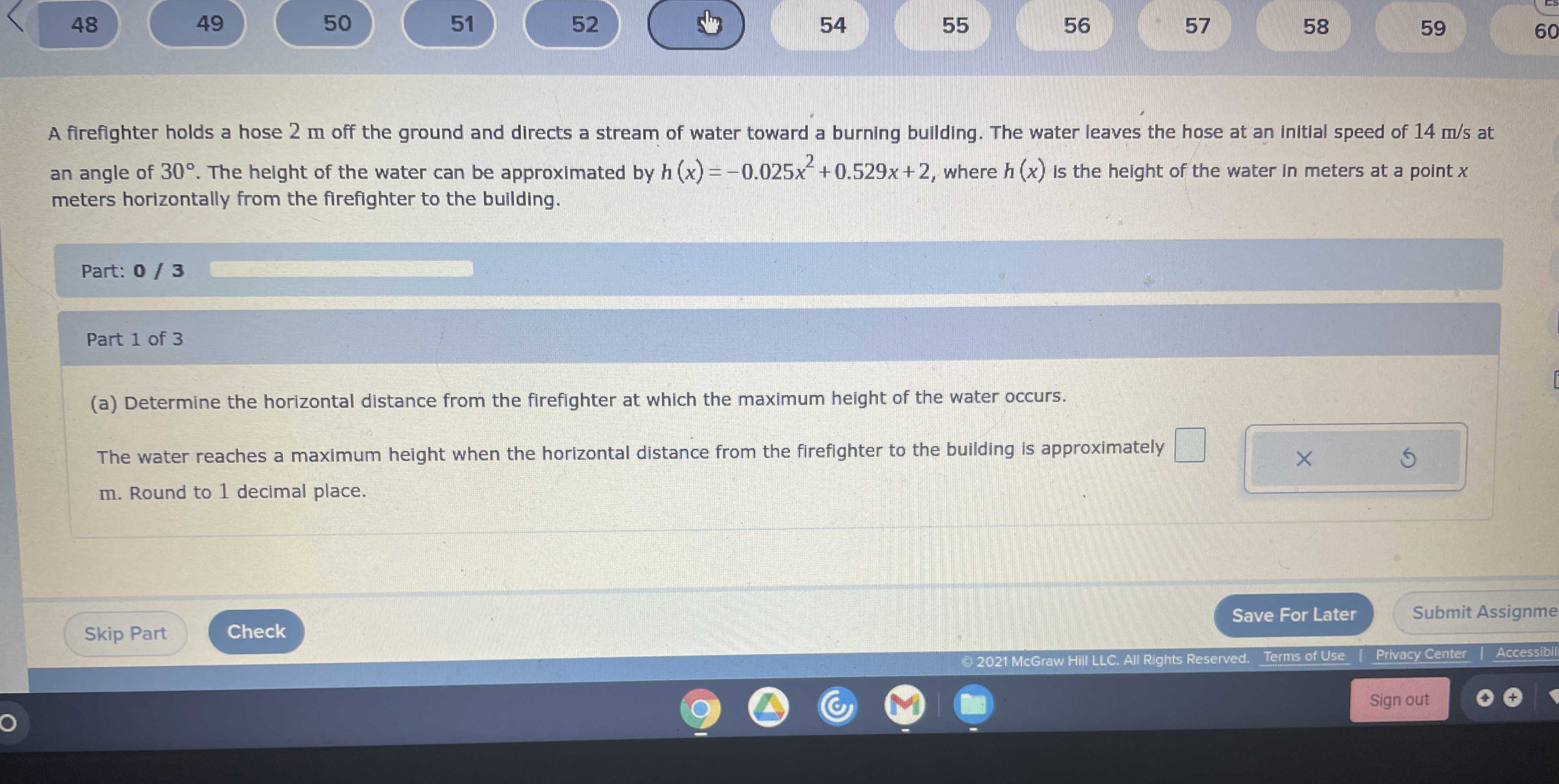### ¿Todavía tienes preguntas de matemáticas?

Pregunte a nuestros tutores expertos
Algebra
PreguntaA firefighter holds a hose $$2 m$$ off the ground and directs a stream of water toward a burning building. The water leaves the hose at an initial speed of $$14 m / s$$ at an angle of $$30 ^ { \circ }$$ . The height of the water can be approximated by $$h ( x ) = - 0.025 x ^ { 2 } + 0.529 x + 2$$ , where $$h ( x )$$ is the height of the water in meters at a point $$x$$ meters horizontally from the firefighter to the building. Part: $$0 / 3$$ (a) Determine the horizontal distance from the firefighter at which the maximum height of the water occurs. The water reaches a maximum height when the horizontal distance from the firefighter to the building is approximately m. Round to $$1$$ decimal place.